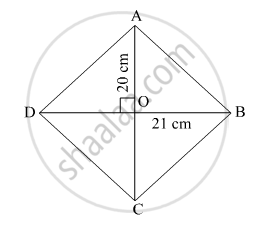Advertisement Remove all ads

# Diagonals of a Rhombus Are 20 Cm and 21 Cm Respectively, Then Find the Side of Rhombus and Its Perimeter. - Geometry

Sum

Diagonals of a rhombus are 20 cm and 21 cm respectively, then find the side of rhombus and its perimeter.

Advertisement Remove all ads

#### SolutionLet ABCD be the rhombus.
AC = 20 cm and BD = 21 cm
We know that the diagonals of a rhombus bisect at right angles.
So, AO = OC = 10 cm
And BO = OD = 10.5 cm

In ΔAOB,

AO^2 + OB^2 = AB^2

=> 10^2 + 10.5 ^2 = AB^2

=> 100 + 110.25 = AB^2

=> AB^2 = 210.25

=> AB = 14.5  "cm"

Thus, each side of rhombus = 14.5 cm
Perimeter = AB + BC + CD + DA
= 14.5 + 14.5 + 14.5 + 14.5
= 58 cm.

Is there an error in this question or solution?
Advertisement Remove all ads

#### APPEARS IN

Balbharati Mathematics 2 Geometry 9th Standard Maharashtra State Board
Chapter 5 Quadrilaterals
Practice Set 5.3 | Q 4 | Page 69
Advertisement Remove all ads
Advertisement Remove all ads
Share
Notifications

View all notifications

Forgot password?